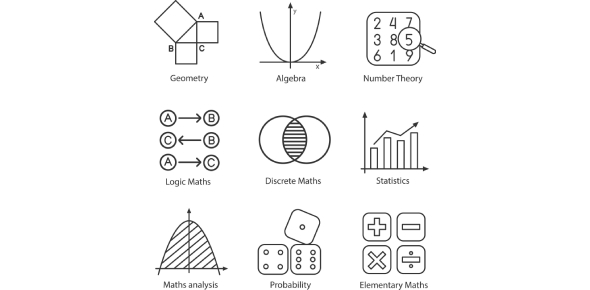# Experiments And Sample Space Quiz

10 Questions | Attempts: 1154
ShareSettingsWould you like to take the "Experiments and sample space quiz?" Experiments and sample space is a random experiment which is a process by which we try to observe something uncertain. After the experiment, the result of some random experiments is known. An outcome is understood as a result of a random experiment. The set of all possible outcomes is the sample space. So, wish you all the best with this quiz. Let's go!

• 1.
Which of the following is an experiment?
• A.

Counting the number of marbles in a jar.

• B.

Measuring the volume of the jar.

• C.

Choosing a marble from the jar.

• D.

Determine the weight of the jar.

• 2.
Which of the following is an outcome?
• A.

Rolling a die.

• B.

Getting a red marble from a jar full of different coloured marbles.

• C.

Flipping a coin.

• D.

None of the above.

• 3.
Which of these outcomes has the highest possibilities with two dices?
• A.

7

• B.

11

• C.

3

• D.

5

• 4.
Which of these is a possible outcome in a dice?
• A.

7

• B.

11

• C.

12

• D.

None of these

• 5.
Two dice are thrown and the values of both dice are added. What is the sample space S?Write the answer in the following format:S = {a, b, c, d, ...., z}
• A.

S = {1, 2, 3, 4, 5, 6, 8, 9, 10, 11, 12}

• B.

S = {2, 3, 4, 5, 6, 7, 8, 9, 10, 11, 12}

• C.

S = {1, 3, 4, 5, 6, 7, 8, 9, 10, 11, 12}

• D.

None of these

• 6.
Outcome is defined as the results of a single trial of the experiment.
• A.

True

• B.

False

• 7.
Which of these cannot be a possible outcome of two dices?
• A.

2

• B.

4

• C.

1

• D.

7

• 8.
An experiment is defined as an outcome.
• A.

True

• B.

False

• 9.
A card is draw from a box containing cards numbered 2, 4, 6, 8, ...., 20 (multiples of 2). Write down the sample space S. What is the total number of possible outcomes, n(S)?
• A.

20

• B.

10

• C.

5

• D.

2

• 10.
Two dice are thrown and the values of both dice are multiplied. What is the total number of possible outcomes n(S)?
• A.

36

• B.

18

• C.

12

• D.

6

## Related TopicsBack to top
×

Wait!
Here's an interesting quiz for you.